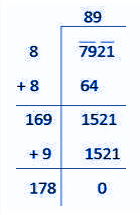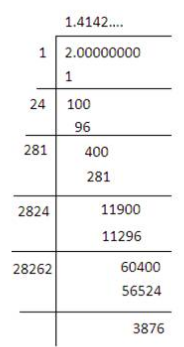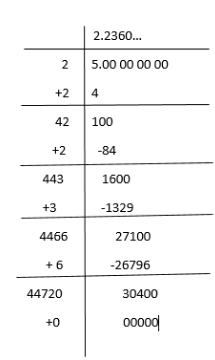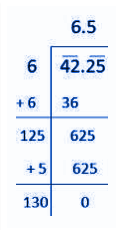# How to Find Square Root

The method to find the square root of any number is easy, if the given number is a perfect square. We can determine the square root of perfect squares by prime factorisation method. But if the number is not a perfect square, then it is difficult to find the square root of it. Hence, we then use long division method.

For example, the square root of 16 is 4, because 16 is a perfect square of 4, such as:

42 = 16 and √16 = 4. But the square root of 3, √3, is not easy, as 3 is not a perfect square.

Let us learn here how to find the square root of numbers which are perfect and imperfect squares.

## Finding Square root By Prime factorisation Method

We can always find the square root of perfect numbers using the prime factorisation method. Let us see some examples here:

1. Square root of 81

Answer: By prime factorisation, we know:

81 = 3 x 3 x 3 x 3

Pairing the numbers to get the perfect squares we get;

81 = 9 x 9 = 92

Hence, √81 = 9

1. Find the square root of 625.

Answer: By prime factorisation, we know:

625 = 5 x 5 x 5 x 5

Pairing the numbers to get the perfect squares we get;

625 = 25 x 25 = 252

Hence, √625 = 25

### How to find square root using long division method

Another method to find the square root of any numbers is long division method. Let us see some examples here:

Example 1: Find square root of 7921

The long division method for √7921 can be found as given below:Hence, √7921 = 89

Since, 7921 is a perfect square, therefore, we can also find using factorisation method.

7921 = 89 x 89

Example 2: Now if we have to find the square root of 2, then it is difficult to find using factorisation method. Hence, we can determine √2 using long division method, as given below:We can proceed further to more decimal places. Here we have derived √2 value upto four places of decimals.

Hence √2 = 1.4142..

Example 3: Find square root of 5 using long division method.Below are the steps explained to find √5:

1. Write number 5 as 5.00000000
2. Take the number whose square is less than 5. Hence, 22 = 4 and 4<5
3. Divide 5 by such that when 2 multiplied by 2 gives 4. Subtract 4 from 5, you will get the answer 1.
4. Take two 0 along with 1 and take the decimal point after 1 in the quotient.
5. Now add 2 in the divisor to make it 4. Take a number next to 4, such that when we multiply with the same as a whole, then it results in the value less than or equal to 100. Hence, 42 x 2 = 84 is less than 100.
6. Now write it below 100 and subtract it from 100 to get the remainder.
7. Next remainder is 16
8. Again carry down two pairs of zero and repeat step 5 up to 4 places of decimals.
9. Finally, you get the answer as 2.2360…
10. You can repeat the method further.

### Square root of Decimal Number

The decimal numbers could be a perfect square or not. But, to find its square root we cannot use factorisation method directly. Let us see an example:

Example: √6.25 =?

Let us write 6.25 as 625/100

Now we know:

625 = 25 x 25

100 = 10 x 10

√625 = 25 and √100 = 10

Thus, √6.25 = √(625/100) = 25/10 = 2.5

Hence, we found the square root of 6.25 equal to 2.5.

Now, if we have to find square root of a decimal number using long division method, then see the example given below:

Example: √42.25 =?Hence, √42.25 = 6.5

### Related Articles

Quiz on Finding the square root